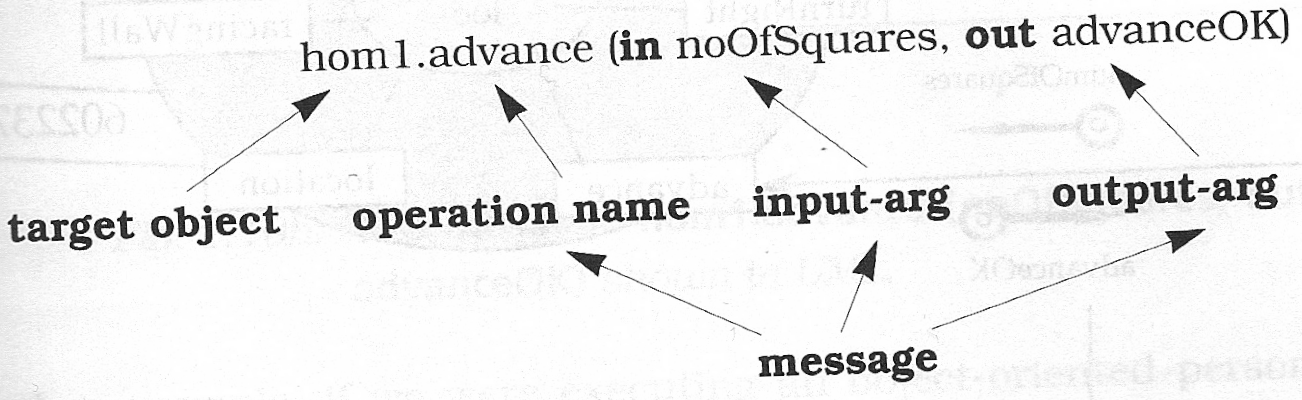## Message arguments

Like functions in traditional programming, messages pass arguments back and forth.

Example of an advance function that has two arguments:

• noOfSquares that tells the function how many numbers of squares to advances (input)

• advanceOk tells the sender if it worked (or if there was a wall) (output)

Figure 6.1. Dissection of a message and its componentsThe same in C++ notation

```hom1->advance(noOfSquares,advanceOk);  // C++ notation with pass-by-reference

Rember: One output value may be done as a return value, multiple output values have to be done with pass-by-reference.

There are 3 types of arguments:

in

input arguments (pass-by-value)

out

output arguments (return value in C++ if only one, otherwise pass-by-reference)

inout

combination of in and out. (pass-by-reference)

If the direction is not shown, and is not clear from the context, it usually means "in"

In UML, in and out can be shown before the parameter name

Note for MagicDraw: In Magicdraw set "show operation parameters direction kind" to true.

Figure 6.2. Hominoid with one operation with in and out parametersIn pure OO languages (smalltalk), all arguments are object handles.

In mixed programming languages (C++, Java) there are two types of arguments

• object handles (in C++: pointers, e.g. Car* )

• data (int, boolean, float, ...)

In UML, messages are shown in "communication diagrams"

We now know 3 types of UML diagrams:

• Class diagrams, contains classes (Chapter 3 in UML distilled). Defines a static view.

• Object diagrams, contains instances (Chapter 6 in UML distilled). (In MagicDraw this is also in class diagram, however in "real application" these should not be mixed). Defines a snapshot view of the system.

• Communication diagrams, contain instances and messages (Chapter 12 in UML distilled), Defines a "dynamic view" of the system.

Generally, these 3 should not be mixed!

Figure 6.3. Call to a Hominoid ObjectIn a communication diagram:

• Instances are shown (Magicdraw does these in green)

• Associations are shown where messages are sent

• A message is an arrow next to an association. The arrow has to be a solid triangle! (this denotes a synchonous call, which is what we'll do in this class)

• The label for the arrow shows:

• The sequence number (in what order do the messages appear?)

• The name of the operation to call

• The input and output arguments, as needed.

Alternate Notation for return values:

Figure 6.4. Call to a Hominoid object with return arrowIn this case, the return values are shown on a return arrow. A return arrow:

• is an open arrow

• is dashed

Sequence numbers describe the order in which the events are sent. Sequence numbers are consecutive (1, 2, 3, 4, 5, ...). They may contain sub-sequences (2, 2.1, 2.2, 3, 4, 4.1, 4.2, 4.3, ...)

Figure 6.5. Timer detonating two bombsMessages from the outside are usually shown with no originator.

Note: MagicDraw does not allow this. We have to use an empty class.

Practice: Assume the following class diagram and the sate given in the following object diagram

Figure 6.6. A TV with a Remote and TunerAssume the user presses the channelUp button on the remote. Show a communication diagram of what happens.

• You will need 3 links (one going to nowhere)

• You will need 5 messages (4 calls + 1 return message)

Possible Solution:

Figure 6.7. Communication diagram for TV